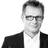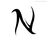How to calculate a sum of a part of data linesHallo, I like to make a sum of all data, which have the same value in a second colume. For example:

Colume one: "Leistung" Values (Beratung / Nebenkosten )

Colume two: "Berechnung" Value = €

I like to install a field which calculate the sum of "Berechnung" if the correspondent field "Leistung" is the value "Beratung".

regards MartinHi Martin,

if the Leistung is choice field and Beratung is the first choice, use formula field with this code:

sum((select YourTable where Leistung = 1).Berechnung)

NickHi Nick,

thanks for your help. I did the same wihtout any positiv result. I think the data structure is the problem. So I will check this point now.

Thanks

Martin

Hallo Martin,

Deine Felder sehen Deutsch aus. Es gibt auch ein deutsches Forum:

https://ninoxdb.de/de/forum/technische-hilfe-5ab8fe445fe2b42b7dd39ee8